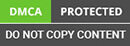﻿ How to Implement Edge Detection Using OpenCV?

Looking for an Expert Development Team? Take two weeks Trial! Try Now

# How to Implement Edge Detection Using OpenCV?In this, we will see how to detect edges in an image. You must be thinking of what is the need of doing that. But there is a lot of requirement just creating outlines, boxes during image processing mainly.In machine learning technology itself, there is a whole lot of requirement while solving some computer vision tasks.

If you heard about a convolutional neural network then it is a huge network and one layer of it as well detect edges must be using some optimized approach. But let’s walk through a simple approach using OpenCV concept of Image gradients

As you must be getting an idea of its application of majorly detecting edges. In image gradients, we calculate the derivative of the whole image matrix in either horizontal or vertical or in both as well.

Calculation of gradient results in a matrix which design an edgy part of the original image.

• ### Sobel

It will find the edges in either of the direction. You can specify the direction of the derivative. You can also specify the kernel size by parameter “ksize”.

• ### Laplacian

It is the combination of Sobel gradient in both direction. We will try to see the python software development of it with the help of some code.

### Mathematical Reason

You must be thinking of how derivatives can help in detecting edges in an image. Let’s dive into the mathematical details of it.

As we calculate the derivative of an image its curve changes as the different color intensity becomes upright.

Let me show you up through image clarification.

I am taking an example of a 1-D image which will be easier to explain.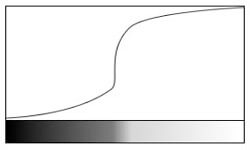As you can see in the above figure, a simple 1-d image with black color has low intensity and white has high intensity. And above that image its graph of intensity pixel values and in derivatives itself we take derivatives of pixel matrix.

So, if will take derivate of the above image it will like as -:A derivative of the above image shows that change in steep means detecting as an edge. But sometimes steeps don’t represent the edge it can be noise as well. Hence, we have to keep a threshold value to detect proper edges.

So, comes Laplacian gradient works on a double derivative. It marks the edge where there are zero crossings of a single derivative and double derivative.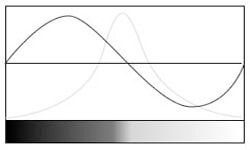So, in the above graph, we have both single and double derivative, and the double derivative is crossing at zero you can see is the point where edge is detected.

### Sobel Detector

They work on single derivatives with some approximation. So, it works separately for horizontal and vertical directions as you can check below in the example.

It chooses a different kernel for a horizontal and vertical detector.

### Summarizing Laplacian and Sobel

Laplacian is computationally efficient as it uses one kernel while Sobel uses two separate kernels. Laplacian works on double derivatives and most precise in detecting edges while Sobel works on single derivative and works on approximation.

### Implementing an algorithm for detecting edges in images

import cv2 import numpy as numpy import matplotlib.pyplot as plt## Reading image img = cv2.imread('images/gradients2.jpg', 0)## Creating a laplacian, sobel##(1, 0) - > horizontal and(0, 1) - > vertical laplacian = cv2.Laplacian(img, cv2.CV_64F) sobelx = cv2.Sobel(img, cv2.CV_64F, 1, 0, ksize = 5) sobely = cv2.Sobel(img, cv2.CV_64F, 0, 1, ksize = 5)## Plotting images## With gray scale images = [img, laplacian, sobelx, sobely] titles = ['Original', 'Laplacian', 'SobelX', 'SobelY'] for i in range(4): plt.subplot(2, 3, (i + 1)) plt.imshow(images[i], cmap = 'gray') plt.title(titles[i]) plt.show()## Without gray scale for i in range(4): plt.subplot(2, 3, (i + 1)) plt.imshow(images[i]) plt.title(titles[i])

Input image is -: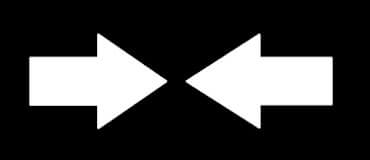Output image is -:Output without gray scale -: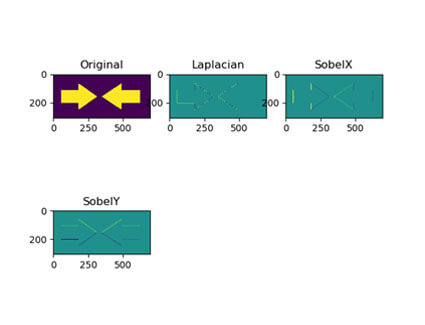### Important Matter

In the above implementation, we have used cv2.CV_64F, but if we change it to cv2.CV_8U then it will give different results with less edge detected.

As, while calculating derivative, transition from black to white is taken as +ve slope while white to black as -ve slope. And CV_8U ignores the -ve derivative part and detect some of the edges. You can try on the above example and test out the output.

### Applications

• Finding boxes in application forms
• Creating features out of which one is edges coordinates for image classification.

### Conclusion

In this tutorial, we have seen how to detect edges in image using OpenCV functions. Keep this point in mind if you are having different version of OpenCV then most probably you will get different kind of images but with same edge detection.

Apart from that, as we have used cv2.CV_64F, there is another function cv2.CV_8U which is not able to detect all the edges try with that as well. There is a mathematical reason behind it that in cv2.CV_8U all the -ve slopes are reduced to 0 and some information is lost.

I hope you enjoy learning! Keep up learning!

### Categories